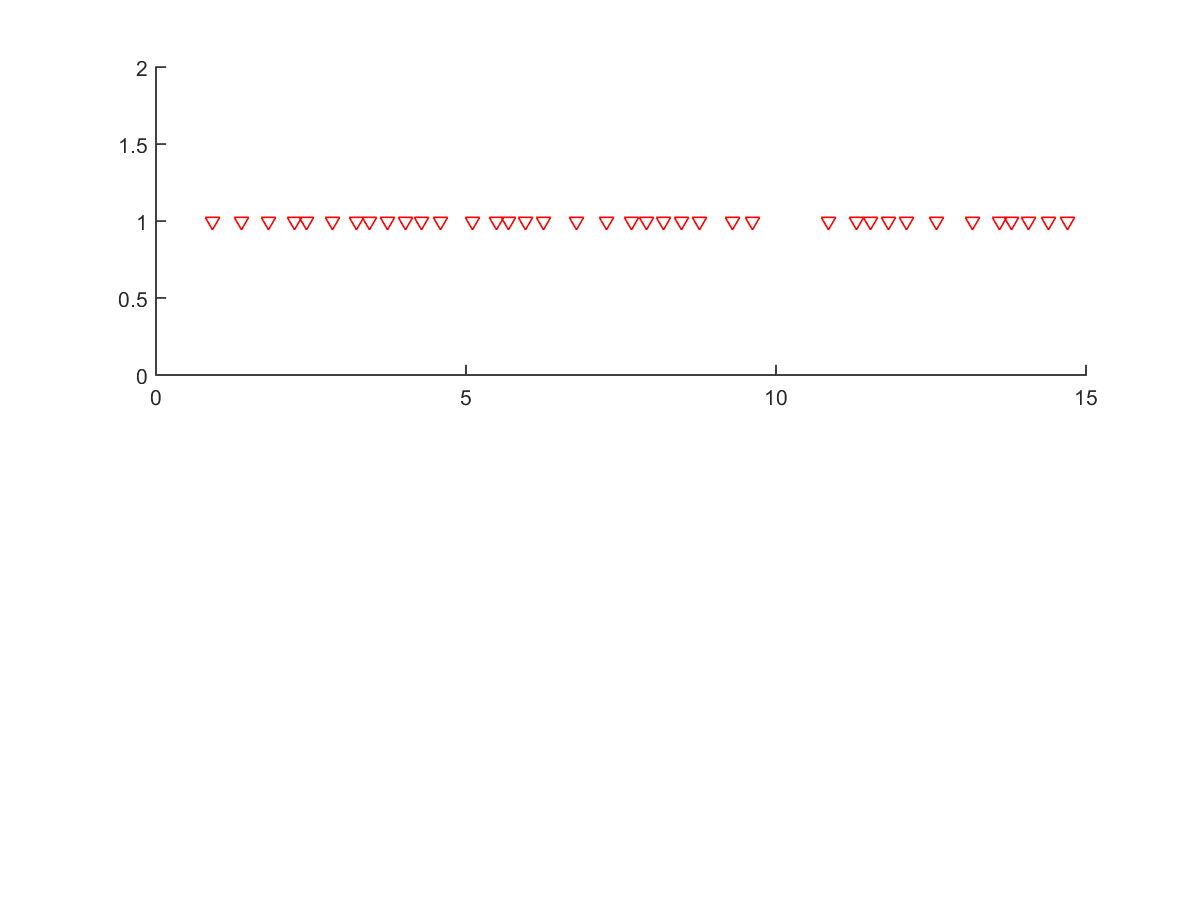## 15-2 Feature Extraction

Similar to other applications of recognition/retrieval, the first step of QBT is feature extraction. Depending on the user interface, there are two types of inputs from the users:
• Symbolic input: The onset time is provided by the user directly. For instance, the user may tap on the keyboard to give the onset time. Such a user interface is easier to design and can be integrated into a webpage to collect the user's input.
• Acoustic input: The onset time is extracted from acoustic input where is generated when the user tap on the microphone. We need to further process the acoustic input in order to extrac the onset time. We shall focus on this type of input for feature extraction in this section.
An intuitive method for extracting the onset time of the tapping is by its volume. A typical example of QBT acoustic input and its volume:

Example 1: qbtVolume01.mwaveFile='tapping.wav'; au=myAudioRead(waveFile); y=au.signal; fs=au.fs; nbits=au.nbits; opt=wave2volume('defaultOpt'); opt.frameSize=320; opt.overlap=304; opt.frame2volumeOpt.method='absSum'; volume=wave2volume(au, opt); % Plotting sampleTime=(1:length(y))/fs; frameTime=frame2sampleIndex(1:length(volume), opt.frameSize, opt.overlap)/fs; subplot(2,1,1); plot(sampleTime, y); xlabel('Time (sec)'); ylabel('Waveform'); subplot(2,1,2); plot(frameTime, volume); xlabel('Time (sec)'); ylabel('Volume');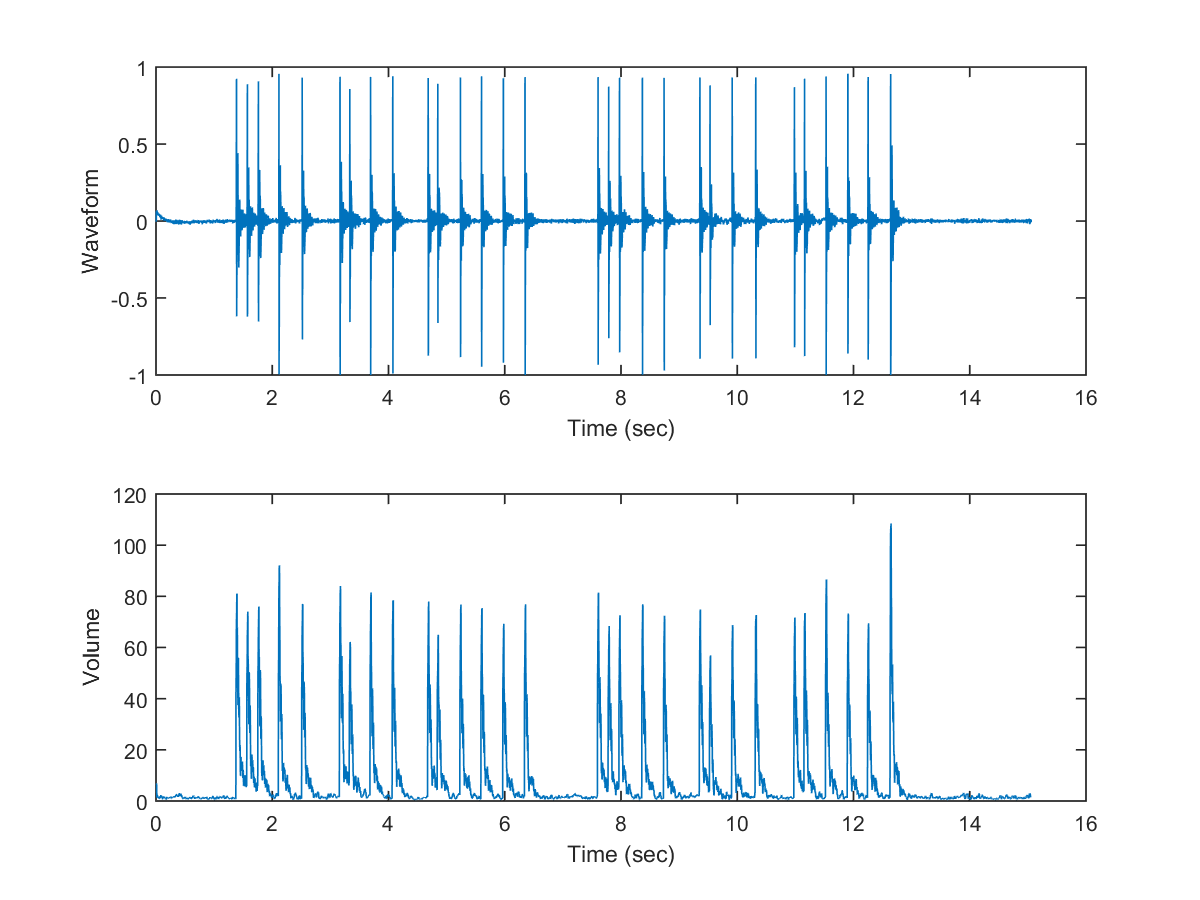Hint
Can you identify the intended song of the tapping?

Hint
Note that for increasing the resolution of the volume, we used a larger overlap such that the frame rate is fs/(frameSize-overlap) = 16000/(320-304) = 1000.

From the above plot, it is obvious that the onset time of each tapping resides at peaks of high volume. One way to extract the onset positions involves the following two steps:

1. An onset position must satisfy two constraints:
• Its volume is greater than a threshold.
• Its volume is a local maximum, that is, greater than its neighbors' volumes.
2. Moreover, an onset position must have the maximum volume within a moving window centered at the onset postion.
The next step demonstrate the result after each of the above steps:

Example 2: qbtFeatureExtract01.mwaveFile='tapping.wav'; au=myAudioRead(waveFile); y=au.signal; fs=au.fs; nbits=au.nbits; opt=wave2volume('defaultOpt'); opt.frameSize=round(fs*0.02); opt.overlap=opt.frameSize-round(fs/1000); opt.frame2volumeOpt.method='absSum'; volRatio=0.5; maxTappingPerSec=10; halfWinWidth=fs/maxTappingPerSec/(opt.frameSize-opt.overlap); % Half width of the moving window % ====== Step 1: Apply a volume threshold to have onset candidates volume=wave2volume(au, opt); frameNum=length(volume); volTh=max(volume)*volRatio; onset1=(volume>volTh) & localMax(volume); % ====== Step 2: Apply a moving window to select the right onset onset2=onset1; index=find(onset2); for i=index startIndex=max(i-halfWinWidth, 1); endIndex=min(i+halfWinWidth, frameNum); [junk, maxIndex]=max(volume(startIndex:endIndex)); if maxIndex+startIndex-1~=i onset2(i)=0; end end onset=frame2sampleIndex(find(onset2), opt.frameSize, opt.overlap); % Plotting sampleTime=(1:length(y))/fs; frameTime=frame2sampleIndex(1:length(volume), opt.frameSize, opt.overlap)/fs; subplot(2,1,1); plot(sampleTime, y); % Display the detected tapping axisLimit=axis; line(onset/fs, axisLimit(3)*ones(length(onset),1), 'color', 'k', 'marker', '^', 'linestyle', 'none'); xlabel('Time (sec)'); ylabel('Waveform'); subplot(2,1,2); plot(frameTime, volume, '.-'); xlabel('Time (sec)'); ylabel('Volume'); line([frameTime(1), frameTime(end)], volTh*[1 1], 'color', 'k'); line(frameTime(onset1), volume(onset1), 'marker', '.', 'color', 'g', 'linestyle', 'none'); line(frameTime(onset2), volume(onset2), 'marker', '^', 'color', 'k', 'linestyle', 'none');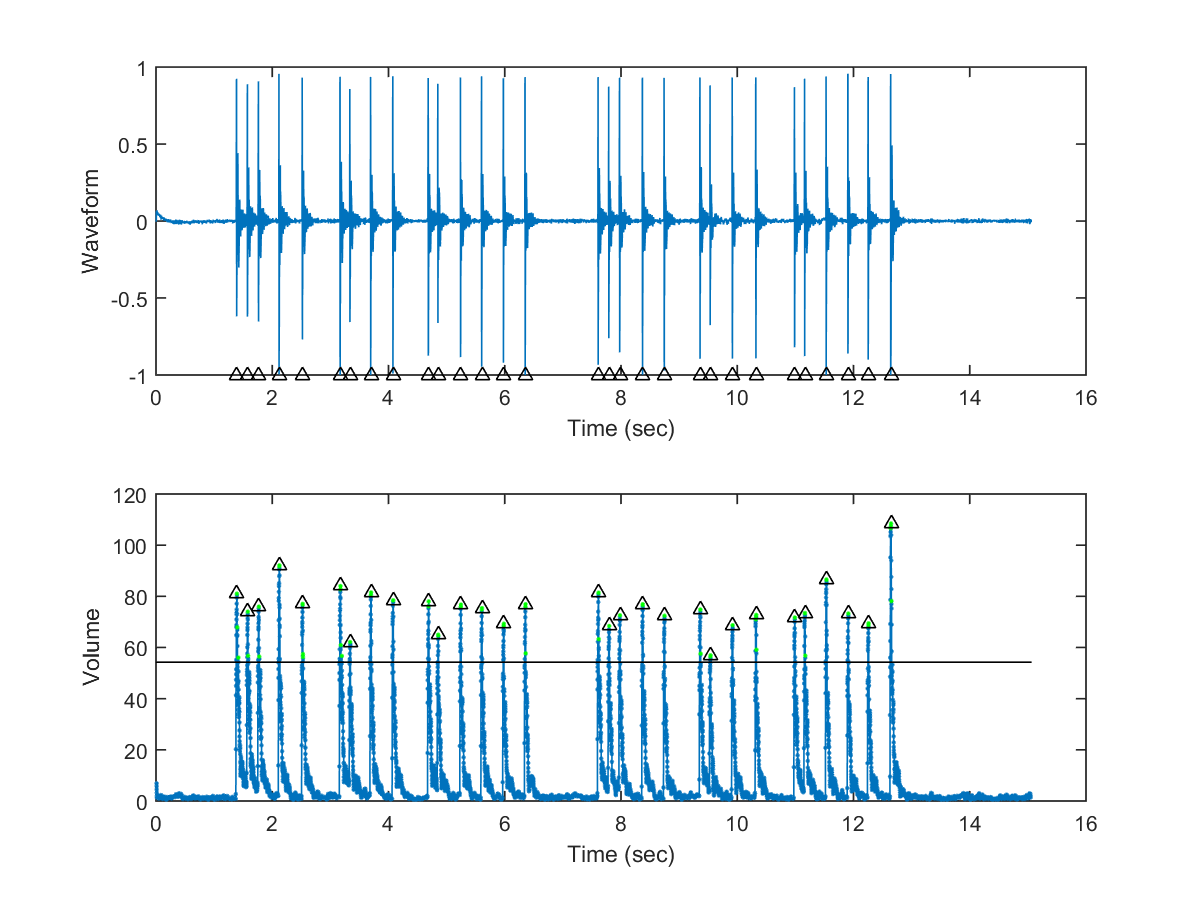In the second plot of the above example:
• Green dots indicate onsets after step 1 of volume thresholding and local-maximum requirement.
• Black triangles indicate onsets after step 2 of the maximum requirement over a moving window.
As can be seen from the plot, these two steps can identify the correct onsets of the tappings. It should be noted that there two parameters in the above procedure for onset detection, the volume ratio and the moving window's width. The volume rato determine the volume threshold, with the following effects:
• If the volume ratio is too small, we will get more onsets with likely insertion errors.
• If the volume ratio is too big, we will get less onsets with possible deletion errors.
In practice, the value of the volume ratio should be determined by a set of training data with ground-truth onsets (which are usually labeled by humans).

The width of the moving window is actually determine by the max tappings rate per second, which is set to 10 in this case. (Can you tap more 10 times in a second? Try your best to see how fast you can tap.)

In fact, we can embedded the labeled onset time in a wav file using CoolEdit. The labeled onset time can be retrieve via wavReadInt.m, as shown in the following example: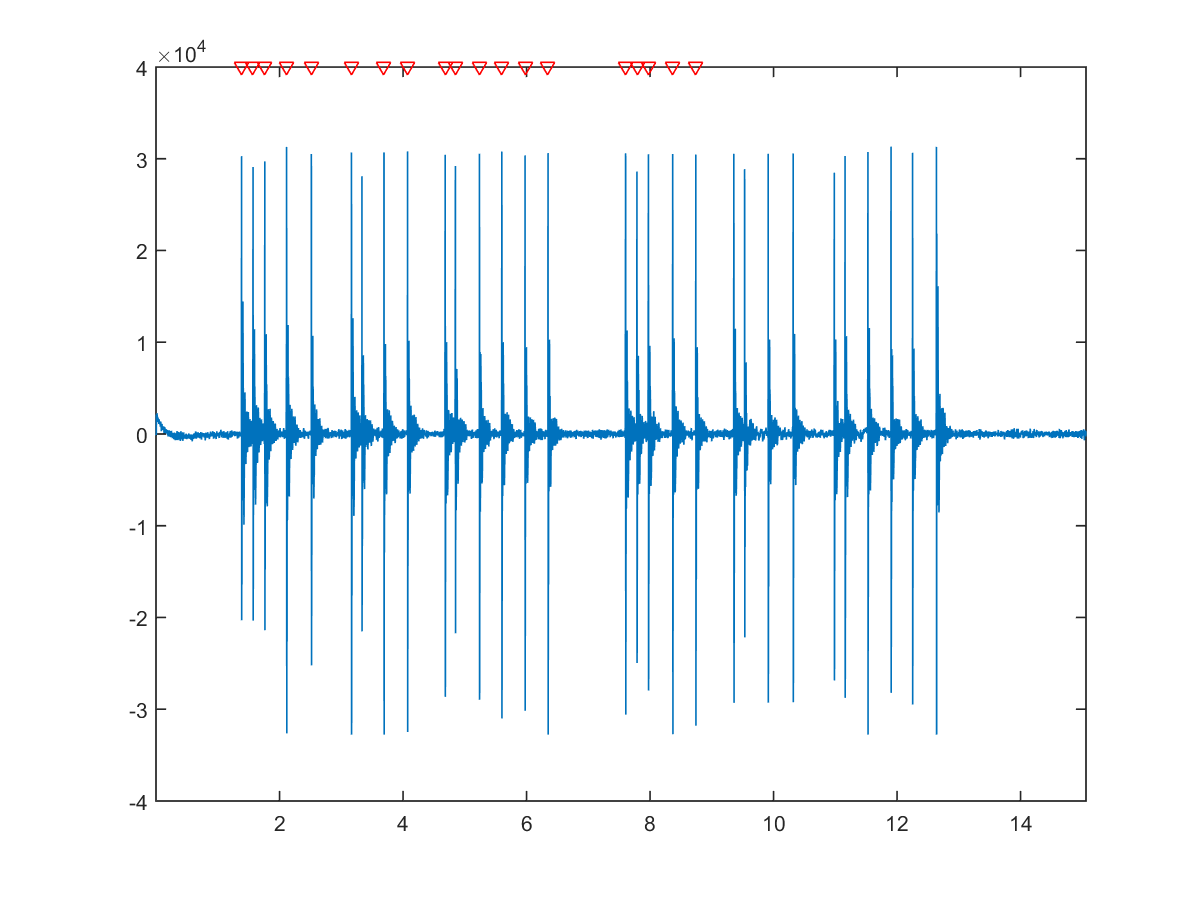We can combine the above examples into a function for tapping detection, as shown next: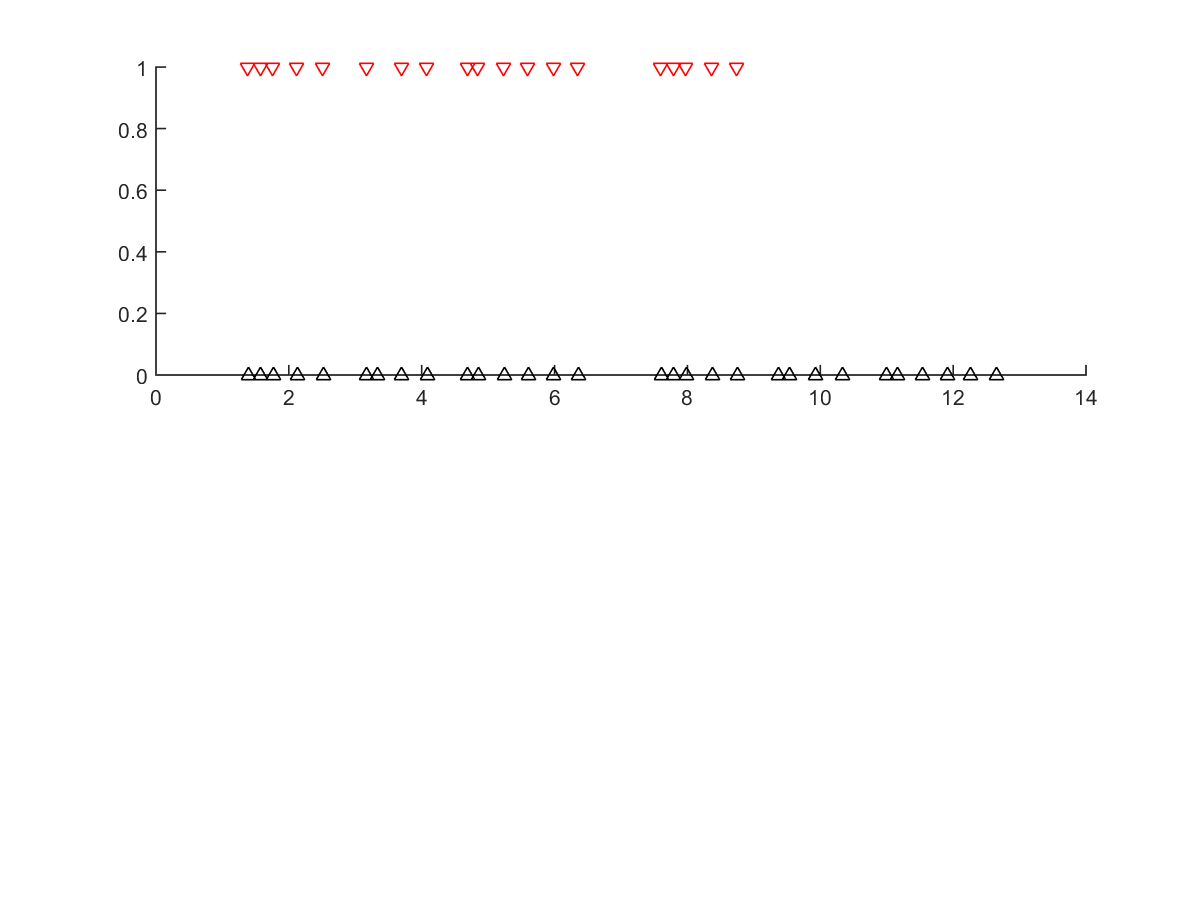Note that in the first plot of the above example, we used black and red triangles to indicate the locations of the detected and human-labeled onsets, respectively.

Since the above example is used quite often to examine the results of onset detection and compare them to the ground-truth, we have compiled another function odByVolViaFile.m for such purpose, as shown in the next example: